# Spin-polarized e-bands¶

This example shows how to plot the band structure of nickel using the eigenvalues stored in the GSR file produced at the end of the GS run.

•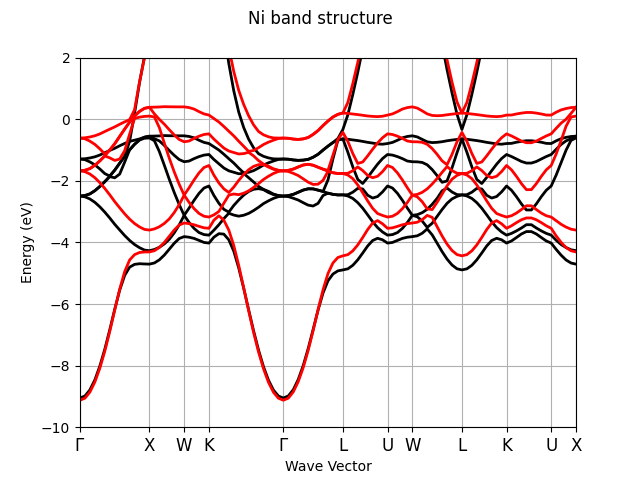•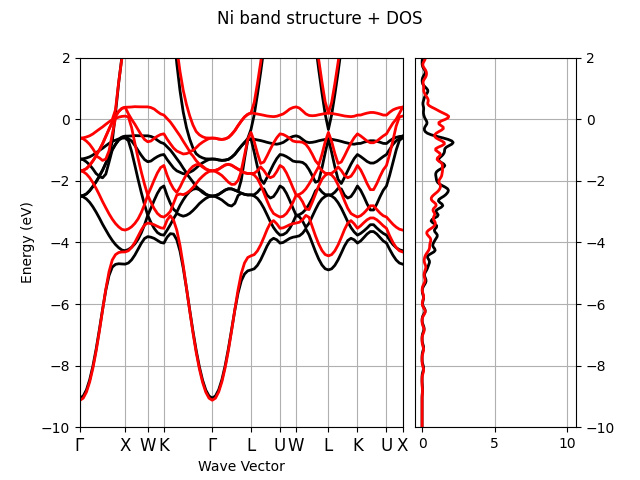•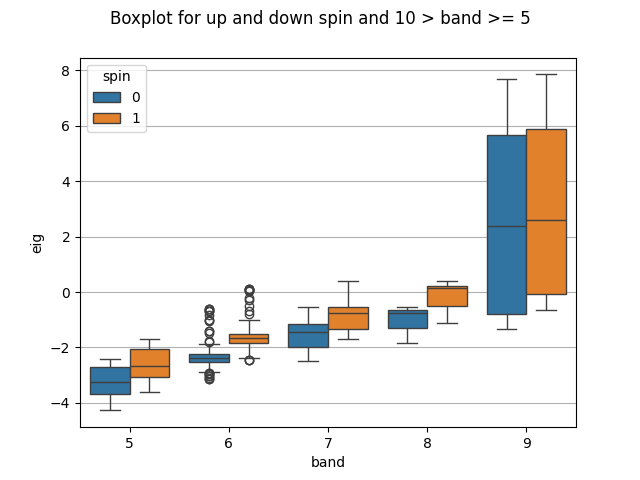•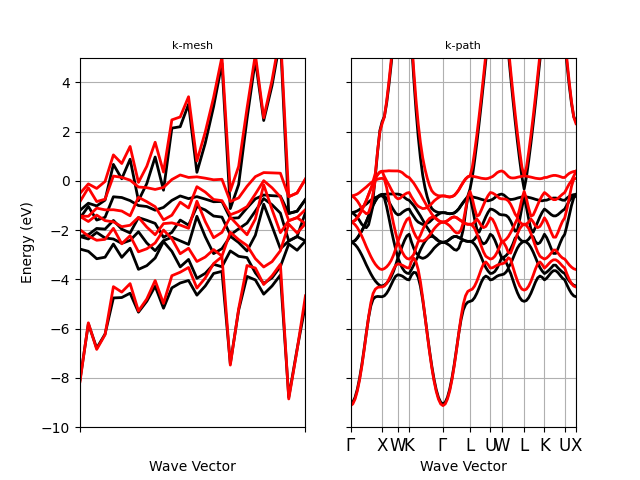•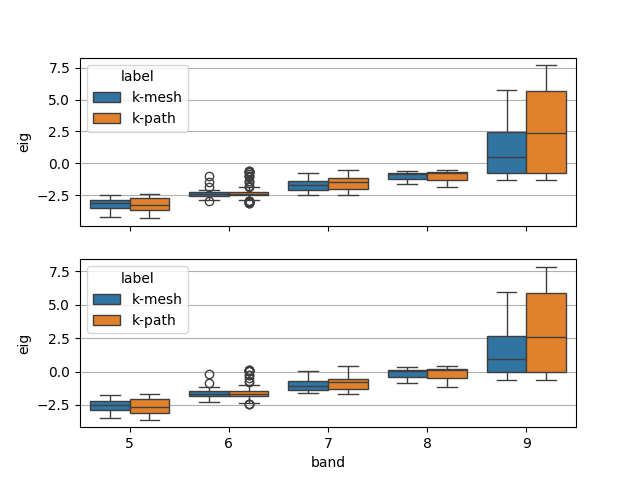Out:

```<Figure size 640x480 with 2 Axes>
```

```from abipy import abilab
import abipy.data as abidata

# Open the GSR file and extract the band structure.
# (alternatively one can use the shell and `abiopen.py OUT_GSR.nc -nb`
# to open the file in a jupyter notebook.
with abilab.abiopen(abidata.ref_file("ni_666k_GSR.nc")) as ncfile:
ni_ebands_kmesh = ncfile.ebands

with abilab.abiopen(abidata.ref_file("ni_kpath_GSR.nc")) as ncfile:
ni_ebands_kpath = ncfile.ebands

# Energy limits in eV for plots. The pseudo contains semi-core states but
# we are not interested in this energy region. Fermi level set to zero.
elims = [-10, 2]

# Plot band energies on k-path
ni_ebands_kpath.plot(ylims=elims, title="Ni band structure")

# Compute DOS with Gaussian method.
ni_edos = ni_ebands_kmesh.get_edos()

# Plot energies on k-path + DOS
ni_ebands_kpath.plot_with_edos(ni_edos, ylims=elims,
title="Ni band structure + DOS")

# Plot electronic DOS.
#ni_edos.plot_dos_idos(xlims=elims)
#ni_edos.plot(xlims=elims)
#ni_edos.plot_up_minus_down(xlims=elims)

# Boxplot for 10 > band >= 5
ni_ebands_kpath.boxplot(brange=[5, 10],
title="Boxplot for up and down spin and 10 > band >= 5")

# Use ElectronBandsPlotter to analyze multiple ElectronBands object
plotter = abilab.ElectronBandsPlotter()